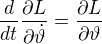## Example for spherical coordinates

One possible transformation from spherical coordinates (r,φ,ϑ) to cartesian coordinates (x,y,z) is:where:where:## Geodetic distance

My current try is:

Lengthis defined to be:So if, then:The usual definition of "distance" is "minimum length", so try to find the minimum:-> minwhere:And x, y, z as above, but with (r,φ,ϑ) being functions of t.

So the extremum is determined by:I'm not sure how to stay ON the sphere with the curve.And so:After dropping the square root (is that safe?), one gets:How to stay on the surface of the sphere?

## Spherical Angles

The Spatial Angle Ω on a sphere with radius R is defined to be:Where A is the area on the surface of the sphere enclosed by beams from the center to the object.

This is so that: